A Detailed Classical Description of the Advance of the Perihelion of Mercury.

P. Marmet, Physics Department, University of Ottawa, Ottawa, Canada K1N 6N5

( Last checked 2018/01/15 - The estate of Paul Marmet )
<><><><><><><><><><><><>
Eine deutsche Übersetzung dieses Artikels finden Sie hier.
Abstract.
Using Einstein's general relativity, it is generally believed that space and time distortions are absolutely required to explain the advance of the perihelion of Mercury. This is untrue. The advance of the perihelion of Mercury was first calculated in 1898 by Paul Gerber (1A). We show here that this phenomenon can be fully explained using Newton's physics and mass-energy conservation, without any relativity principle. Without having to introduce any new physics, we arrive to the same equation as predicted by Einstein. Therefore, the relativity principles are useless.

1- Introduction.
A preliminary classical demonstration of the advance of the perihelion of Mercury has been given previously in the book: Einstein’s Theory of Relativity versus Classical Mechanics, (chapters 5 and 6), ,without using any of Einstein's postulates. That demonstration uses mathematical relationships, which are based on classical mechanics and mass-energy conservation. We explain now in detail, all the physical mechanisms involved in the calculation of the advance of the perihelion of Mercury. Using only classical mechanics, we obtain the same equation as predicted by Einstein’s General Relativity, without using any of the relativity principles. We have seen  that the advance of the perihelion is due to gravitational potential and kinetic energy, which modify the standard meter and the clock rate on Mercury. The physical change of length and the change of clock rate were demonstrated previously  .  The only difference between the usual Newton’s physics and the results presented here is that, contrarily to Einstein, we fully take into account the principle of mass-energy conservation. This paper shows how to use the units existing on Mercury to derive the correct shape of the precessing elliptical orbit around the sun.

2- Orbital Motion.
An ellipse with a small eccentricity is identical to a circular orbit, in which the center of the circle has been slightly shifted. This can be seen more easily when we examine in detail the transformations of shapes from a circle to an ellipse. This geometrical transformation is demonstrated in Appendix 1. However, when a circle is slightly perturbed to become an ellipse, the change of shape is usually described by the gradual transformation from a circle to the familiar elongated characteristic shape of an ellipse. In the case of the elliptical shape of an orbit around the sun, since the eccentricity is small, this is equivalent to a circle with a shifted center, because in fact, when adding a small eccentricity, the first mathematical term of the series expansion of an ellipse appears as a shift of the central circular field of forces.  It is only the second term of the series expansion of an ellipse, which flattens the orbit into the well-known elongated shape (see Appendix1). This description is useful for a better understanding of the physics discussed here.
Let us consider a mass m orbiting the sun. Newton’s laws show that, due to the centrifugal force, when the orbiting velocity is such that the radius of curvature of the orbiting mass is compatible with distance of a circular orbit around the sun, that radius of curvature is stable.  Such a circular orbit is illustrated around the shaded area on figure 1. In that case, the moving mass is always at the same constant distance ro from the sun.Figure 1

Consequently, in a circular orbit, the mass is continuously subjected to a constant gravitational force, which is always exactly compensated by an equal constant centrifugal force everywhere on the orbit. The centrifugal force F(centri) and the gravitational force F(grav) are exactly equal and opposite, so that their sum is everywhere zero at the distance ro from the sun. We have:1
In this paper, only a single plane of the orbits needs to be considered.
Let us consider that at the distance ro from the sun, the curved trajectory represents a curved axis of coordinates (the curved horizontal axis on figure 2) along the circular trajectory around the sun. Planet Mercury travels at a constant velocity V along that curved axis. At radii r+Dr and r-Dr, (dashed circles on figure 1), the corresponding (dashed) parallel lines to the ro axis are drawn on figure 2.  Of course, there exists no force F accelerating the mass along this tangential direction, since the centrifugal force compensates for the gravitational force at ro.  This axis is perpendicular to a radial r-axis (drawn vertically on figure 2).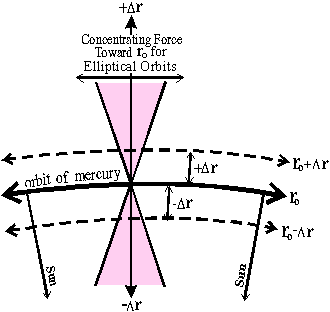Figure 2
Since we have seen in equation 1 that the two forces cancel out at a distance ro, there remains no net force Fr, in the radial direction, as long as the distance of the mass from the sun remains ro
If, at one point on the orbit, the mass is deflected toward an outer direction (illustrated at "m" on figure 1), its distance r from the sun increases. However, its velocity will then gradually decrease because of its increasing gravitational potential in the new direction further away from the Sun. Therefore, the slower particle will gradually have a "radius of curvature" (around the aphelion in A, on figure 1) smaller than its distance (radius r+DR) from the Sun. This will then make the mass fall back to a closer distance to the Sun. Then, when approaching the perihelion B, inside the initial orbit ro, the velocity will then increase so that the radius of curvature will now be larger than its distance from the Sun. This phenomenon is repeated periodically at every rotation around the sun.
This qualitative description shows that in such an elliptical motion, the mass oscillates (along the radial r-axis) from one side to the other of the average radius ro, as shown in dotted (not dashed) line on figure 1. Since the mass oscillates on each side of the average radius ro, there must exists a net force, always pulling it in the opposite direction, always toward ro. Let us calculate this force.

3 - Constant Curvature of an Ellipse with a Small Eccentricity.
We can see in Appendix I, a geometrical demonstration showing that an ellipse with a small eccentricity (first order) is identical to a circle in which the center has been shifted. Therefore such an ellipse is a circle with a displaced center (first order). Let us demonstrate now, that the laws of physics also lead to a constant radius of curvature R when a mass travels on an elliptical orbit (with a small eccentricity). This means that the forces acting on the mass orbiting on an ellipse, and the velocity must be compatible with the constant (radius of) curvature R of the ellipse.
Let us define the "radius of curvature" at one point on the ellipse equal to R, while the distance from the sun is r. Therefore, R is the distance from a point on the ellipse to the center of the ellipse (point "C" on figure 7).  Since an ellipse with a small eccentricity is a circle with a radius R, the centrifugal force must always lead to a constant radius of curvature R. We have:2
The radius of curvature R must remain constant even when the distance r from the sun increases by r. Equation 2 gives:3
Since we have an ellipse, the distance r from the Sun is a variable so that the gravitational force F changes. Furthermore, the velocity V on the orbit changes at the same time. Let us calculate an assumed variation of the radius of curvature R (if any) as a function of the gravitational force F and the velocity V of the orbiting mass. The derivative of equation 3 is:4
This is equivalent to:5
The gravitational force F is equal to: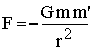6
Its derivative is:7
From equations 6 and 7 we find:8
Let us substitute 8 in 5, we find: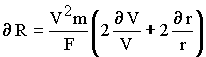9
Since the velocity of the orbiting mass changes when changing its distance from the Sun, let us apply the principle of energy conservation. When r is changing, the variation of potential energy is equal (minus) to the variation of kinetic energy. We have:10
This gives:11
or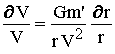12
It is well known in classical mechanics, that in a orbital motion around a central field, the kinetic energy of an orbiting mass is always (minus) half of the potential energy.  Since this is well known, this will not be repeated here.  This can be written:13
This gives:14
Substituting 14 in 12 gives:15
Equation 15 in 9 gives:16
Equation 16 can also be written: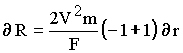which is17
Equation 17 shows that a change dr of distance r from the Sun (in the neighborhood of ro), does not change the radius of curvature R on any point on the elliptical orbit.  Since in equation 17 dR equals zero, this means that in equation 5, the relative change of velocity dV/V just cancels out the effect due to the change of gravitational force dF/F. Therefore the elliptical orbit maintains everywhere the constant curvature R, even if the distance from the Sun is variable. This agrees with a circle with a displaced center as illustrated on figure 1 (also in Appendix I).

4 - Spring Constant
On figure 1 and 2, we see a larger circular orbit at (ro+Dr) and a smaller circular orbit at (ro-Dr) illustrated by dashed lines, while the elliptical orbit of Mercury is illustrated on figure 1 using a dotted line. We can see (especially on figure 2), that those circular trajectories at ro+Dr (and ro-Dr) are parallel to ro (at A and B).  When the mass is located in A, we see (figure 1) that the elliptical orbit does not remain at a constant distance from the original circular orbit (solid line). In order to remain at a constant distance, the particle (on the elliptical orbit) would have to continue along the dashed circular orbit having a radius ro+Dr. Therefore, with respect to this circular orbit, there is a force on the elliptical orbit, bringing the mass back toward the radius ro, when the particle is A.
Similarly, when the elliptical orbit passes at the perihelion, at point B, inside the initial orbit ro, there must be a force pushing the mass back externally, toward the initial radius ro. That force (pushing the mass back toward ro), that exists for the elliptical orbit does not appear for the circular orbit at ro-Dr. Let us calculate that force (always pushing the particle back to the radius ro).
It is clear that the force pushing the particle back to the radius ro at a distance ro±Dr, is equal to the difference of force between the circular orbit and the elliptical orbit passing by A or B. Both the elliptical and the circular orbits pass through a common point at A, (where of course the gravitational force is the same).  Keeping everything else (i.e. energy, velocity and potential) constant, let us calculate the change of force necessary at A, so that the radius of curvature of the mass in the elliptical orbit become equal to the radius of curvature of the circular orbit. This is the force we are looking for in order to make the two orbits parallel in A. It is that difference of force that brings the mass from the distance ro+Dr back to ro (after a quarter of rotation).
In the case of the circular orbit having a constant radius ro, the gravitational force is equal to the centrifugal force so that the sum of the forces is zero.  We have:18
From equation 18, for the circular orbit ro, we have:19
The reason for which the mass traveling on the ellipse at location A (figure 1), moves back to the orbit (toward the average radius ro) is because its velocity had decreased previously, when the distance increased from ro to ro+Dr.  This is a consequence of conversion of the total kinetic energy into gravitational energy.  When the ellipse passes at location A, the distance from the Sun is ro+Dr.
Let us calculate the change of force on the orbiting mass, as a function of the distance from the Sun.  There are two variables, v and r.  The derivative of equation 18 gives the change of force as a function of the radius r and the velocity v.  We get: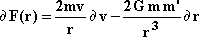20
At location A, the gravitational potential has increased.  Therefore the velocity v must decrease.  Knowing that the total energy is conserved, let us calculate the change of velocity as a function of the distance r from the Sun.  The relationship between the kinetic energy and the potential energy is:21
Let us calculate the derivative of equation 21.  The substitution of equation 21 in its derivative, gives the relationship between the change of velocity with respect to a change of radius when energy is conserved.  Equation 21 and its derivative give:22
Therefore, the change of force calculated in equation 20 can now be expressed as a function of one unique variable dr.  Equation 22 in 20, and then substituting equation 19, we get: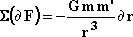23
Equation 23 gives the change of forces bringing the mass back toward ro. This is exactly the force involved in the elliptical orbit passing through location A. This extra force would transform the large circular orbit in A into the elliptical orbit as illustrated on figure 2. Similarly, we find that the same extra force must be applied in the opposite radial direction at location B to bring the particle back to the initial external radius ro (also illustrated on figure 2 and at location B on figure 1). Then in that case, Dro is negative. Consequently, this shows mathematically, that the mechanism of translation of a mass around the Sun is such, that for an ellipse, with respect to the corresponding circle, there is a force bringing the mass back to the initial ro every time a mass is deflected from its initial orbit.   Whether the mass moving on the elliptical path is "inside" or "outside" the circle, the force always push the mass back toward the circle ro.  Furthermore, this force is proportional to the distance dr, as calculated above (equation 23). We must notice that this force has the same mathematical form as the force of elasticity known as Hooke's law. This force is responsible for the elliptical shape of planetary orbits.

5 - Harmonic Oscillator.
What is the behavior of a mass submitted to such a force, which always brings back the mass toward the opposite side of the average radius? According to equation 23, that force focuses toward ro, all orbiting masses, with a force which is proportional to the width of the shaded area illustrated on figure 2. In classical mechanics, that force is characterized by the constant k, (called the spring constant). It is a force per unit of displacement (along the r-axis). The force of a harmonic oscillator  is defined by the relationship:24
In classical mechanics, we know that in order to produce a periodic oscillation across ro, the value of k must be positive, just as obtained above. This problem, well known in physics, has been solved centuries ago and is recalled in numerous textbooks . We know that a force is the second derivative with respect to the distance r. We have:25
Equations 24 and 25 give: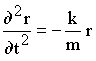26
The solution of equation 26 is a sine wave, oscillating on each side of the central position ro. The period of oscillation P(osc) is:27
Substituting the spring constant k existing on our elliptical orbit as given above, equation 23 into 27 gives: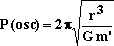28
Equation 28 gives the period of oscillation P(osc) of the mass on each side of the radius ro along a curve axis. It is very important to notice that equation 28 has been obtained independently of the time taken by the mass to complete a revolution of 360 degrees around the Sun. Equation 28 gives the period to complete one full oscillation across either side of the average radius ro. That period of oscillation depends on the spring constant k. It is completely independent of the velocity of the mass along the orbit and of an assumed length of a trajectory. However, the period of rotation (2p radians) of Mercury around the Sun at the distance ro, depends on the circumference of that orbit and the velocity of the mass on that trajectory. It is completely independent of the period found above using the spring constant k.

6 - Comparing two Independent Periods; P(osc) and P(rot).
Let us now compare the time P(osc) taken by the mass to complete one full oscillation on either side of the average radius ro of a circla, and the time P(rot) taken by the same mass to complete one full rotation around the Sun. In the calculation of P(osc), we use the relationship (equation 28), giving the period of oscillation of the particle across the radius ro. Let us transform the parameters under the square root (in equation 28) as a function of the velocity V of the mass moving around the Sun. We know that the gravitational force Fg around the Sun is equal to the centrifugal force Fc. We have:29
where rs is the radius of the orbiting distance of the mass from the Sun.
Equation (29) gives30
Equation 30 in 28 gives:31
Equation 31 is the period of oscillation P(osc) of the mass on either sides of the average radius ro. However, the period of rotation P(rot) of Mercury around the Sun can then be calculated independently, using a simple geometrical relationship. From the relationship using the perimeter of the trajectory divided by the velocity, we get: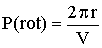32
Equation 32 uses the circumference of a circle equal to the perimeter of an ellipse having a small eccentricity. This is demonstrated in appendix I of this paper. Consequently, when we do not apply any correction for mass-energy conservation, as done usually with Newton's equations, we find that equations 31 and 32 show an extraordinary coincidence between the period of oscillation P(osc) of a mass across the average radius ro and the period of rotation P(rot) of the same mass around the Sun. We have: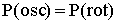33
Let us notice that such a coincidence would not exist if the gravitational field around the Sun would not decrease exactly as an inverse quadratic function. This has been shown in more detail previously . A difference would appear in equations 23 and this would not lead to an equal phase relationship between the periods of oscillation and rotation. This is well known mathematically. Since these two independent periods P(osc) and P(rot) are identical for the inverse quadratic force existing around the Sun, the period of rotation around the Sun coincides with the period of oscillation of the body across the average radius ro as required by Kepler's laws and observed experimentally. Therefore, the major and minor axes of the ellipse are always pointing in a constant direction in space as a function of time. The equality of the period between the oscillation on both sides of the radius and the rotation around the Sun is illustrated on figure 3. Both periods P(osc) and P(rot) are the same.Figure 3

The result above has been obtained using the conservation of potential plus kinetic energy but without mass-energy-conservation.
When energy (kinetic or potential) is given to a mass, the mass of that energy is given to the mass having received that energy, following the relationship E=mc2 as demonstrated previously.  Let us now take into account the principle of mass-energy conservation.

7 - Fundamental Considerations.
In physics, a natural description of the period of rotation is given as the time taken by a body to complete one full rotation by 360 degrees or 2p radians around the Sun. We will see now that Newton's laws do not lead to a perfect ellipse (without precession) when the principle of mass-energy conservation is strictly applied. The fundamental reasons have been explained in the book entitled Einstein's Theory of Relativity versus Classical Mechanics . The full explanations and calculations are too long to be repeated here. You find them explained in detail in the book   but they are also available on the internet. Only a concise description of the fundamental principles is recalled here using some examples. The study of the original paper   is indispensable for the complete understanding of the phenomenon.
Let us mention first that the principle of mass-energy conservation is one of the most important fundamental principles in physics. This principle is always valid and must never be neglected and transgressed. This principle implies that we cannot create from nothing and we cannot annihilate into nothing. Mass and energy are two different aspects of the same constituent of matter. Energy (E) always possesses mass (M) and mass always possesses energy. Mass can be transformed into energy and vice versa. The ratio of proportionality between mass and energy is a constant, which happens to be equal to the square of the velocity of light.
Serious consderations in physics shows that when kinetic or gravitational energy is given to a frame of reference, that increase of energy increases the mass of the frame, corresponding to the amount of energy given to the frame, according to E=mc2.  Whatever the system of reference used in the energetic frame to determne the standard references, we have seen that the local reference standards of mass, length and clock rate change during the passage from rest to the energetic frame.  However, that change of local reference standards are undetectable locally in the moving frame, because matter forming the frame is submitted to the same increase of energy as any other matter used to do experiments inside the moving frame.  Therefore, after the acceleration of both, a frame and all local matter used to do a local experiment, have increased their energy, and therefore their mass.  Therefore, locally inside the frame, this is undetectable.  This is required by the principle of mass-energy conservation "between frames".
The calculation of the Bohr atom, involving quantum mechanics is also requires in that claculation, as demonstrated previously in the book: Einstein's Theory of Relativity versus Quantum Mechanics.  For example, let us consider a grain of sand in space moving around the Sun at the Mercury distance, having a mass of one million atoms. When this grain of sand is moved from Mercury orbit around the Sun to the Earth's distance from the Sun (or about an infinite distance), we need extra energy to move it against the gravitational potential of the Sun. The extra energy can be obtained at the cost of transforming some mass into energy. Suppose that, to provide the required energy to raise the grain of sand from Mercury orbit, to Earth's distance from the Sun, it is necessary to transform one atom of mass "m" into energy (E=mc2). We also assume that that extra energy (extra atom) is taken from an external source near Mercury's orbit. Therefore the total number of atoms leaving Mercury's orbit around the Sun is 1 000 000 (plus the equivalent energy of another one). Then, while the grain of sand is moved away from the Sun, the energy of the disintegrated atom is used up gradually (like "dissolved" into the mass of the grain of sand), so that finally, the number of atoms reaching the Earth distance is again one million, after having transformed the energy of the atom into gravitational (potential) energy.
Then the total number of atoms is the same at Mercury orbit from the Sun (which is 1000000 Mercury atoms plus energy) as at Earth orbit from the Sun (which is agan 1 000000 Earth atoms). Therefore 1000001 atoms at Mercury orbit has the same total mass-energy  as one million atoms at Earth orbit. The mass-energy produced from the disintegration of one atom has been given to all other atoms. The logical explanation implies that each atom has an extra mass-energy after reaching the Earth orbit.  They have a slightly larger mass than the atoms at a lower potential energy on Mercury orbit.  There is no other way to satisfy the principle of mass-energy conservation.
One can see that the same relative change of mass-energy must also exist on the standard reference kilogram used on Earth's orbit from the Sun. The standard reference kilogram can be defined by an absolute number of atoms. The standard kilogram simply contains a much larger number of atoms than a grain of sand. The principle of mass-energy conservation requires that one Mercury-kilogram (at Mercury distance from the Sun) contains slightly less mass-energy than the Earth-kilogram (at Earth distance from the Sun), even if the number of atoms is exactly the same. That change of mass of each atom is real. It is not an illusion. This is required by the principle of mass-energy conservation.
There are several other logical consequences to that change of mass of bodies, due to gravitational or kinetic energies. Since the real physical mass of bodies changes when we add energy, one must realize that particles, like electrons and protons of atoms, forming those masses must logically also change their mass, exactly in the same proportion as atoms.  Furthermore, using quantum mechanics , one can show that a change of electron and proton mass modifies the length of the Bohr radius. Due to that change of the Bohr radius, the physical lengths of bodies and the energy of the quantum levels change when gravitational or kinetic energy is added to a mass. All these phenomena have been explained and calculated previously .
Following the change of the Bohr radius, quantum mechanics also predicts a change of quantum levels, due to the change of electron mass, implying also a corresponding change of rate of atomic clocks  .  It is also required that all matter, including organic matter and even human bodies, function at a different rate when electrons forming them have acquired or released some potential or kinetic energies.  Since Mercury in its orbit has a different gravitational energy and possesses a different kinetic energy, matter on Mercury (i.e. due to its Mercury distance from the Sun) has a different mass. In addition, clocks on Mercury are functioning at a different rate due to the change of electron mass.
However, we have seen above that that change of mass, length and clock rate is undetectable, because matter from this frame, that  forms the standards of reference in a frame, changes in the same proportion as the local matter being investigated inside the same frame.  Consequenlty, the experimental parameters (number of units) measured in the energetic frame are identical to the ones in the initial frame, but they are not coherent with the increase of mass-energy between the two systems of reference.   Since the increase of mass-energy in the energetic frame is real, the numerical values measured inside the energetic frame are not compatible with the principle of mass-energy conservation and therefore are in error.  The relative size of the standard references must be calculated.  The relationships transforming the standard units between locations at different gravitational potentials and different velocities have already been calculated  .  The length of the radius of the orbit of Mercury is a number which is equal to the number of Mercury-meters times the length of the local standard Mercury-meter. However, that number is not equal to the number of Earth-meters to measure the same orbit of Mercury, because it is measured using the shorter Earth-meter.  We must notice that Newton's laws of physics deals with the numbers that are fed into the equations. Since the number of meters to measure the "same physical length" (using the longer Mercury meters) is smaller than the number of Earth meters, we must take that difference into account.
In physics, there exist several systems of units using meters, feet, kilograms, pounds, coulombs, statcoulombs, abcoulombs etc. that have been devised in a coherent way so that the coherent use of any set of reference units leads to answers which are compatible, independently of any system of units. In fact, one has a complete choice of systems of reference units that leads to the same "physical" answer, although represented by different numbers when using units having different names. However, contrarily to the above, when we apply the principle of mass-energy conservation between frames, the units of mass, energy, lengths and clock rates in different frames cannot be the same.  Most importantly, the principle of mass-energy conservation must be satisfied.  The correct calculation requires coherence between frame.  Therefore the local number of units must be corrected with respect to the Earth value considered at infinity. We show here below, that this logical correction explains perfectly the advance of the perihelion of Mercury without any relativity principle.
In the calculation below, for simplicity, we make the approximation that the Earth is infinitely remote from the Sun.  Therefore we consider that the Earth is located in Outer Space.  The number of meters of the Earth from the Sun is noted "NO.S. ".   Corrections due to the residual Earth gravitational potential can be easily done later. Furthermore, when we say that a mass is near Mercury, one must understand that it at a location near the Mercury orbit, assuming that the gravitational energy due to Mercury is zero.
As explained previously , since the number of local units corresponding to local standards of length, mass and clock rates are numerically different in different frames, (due to mass-energy conservation) these different reference standards must be clearly identified without ambiguity. A special notation must identify clearly in what frame the unit is calculated. Our notation uses a sub index to refer to the proper units in use. Notation [meterM] represents the "physical" length of the standard Mercury meter, and [meterE] is the "physical" length of the Earth meter. Therefore these "physical" lenghts are local "etalons".  Also, [N-LengthM], is the number of Mercury meters corresponding to the physical length [LM], and [N-LengthE] is the number of Earth meters corresponding to the physical length [LE]. For example, the physical length (in Earth meters) of the radius of the orbit of Earth is:34
Using Mercury meters, the same absolute physical length (distance) is given by:35
Of course, when we measure the same "realistic physical quantity", we have:36
In the above description, there is no non-realistic concept like "space contraction". There is just a real physical change of length of matter due to the change of the Bohr radius, required by the principle of mass-energy conservation.
Equations 34 and 35 can be used to measure any object located on Earth, on Mercury or anywhere else having a different kinetic energy or gravitational potential. Applying mass-energy conservation, we have explained previously (Chapter 5, equation 5.31 of )¸ that the relationship between the Bohr radius of the atoms of the Mercury-meter [meterM,v,] having a velocity v, and the atoms of the "Outer Space" meter [meterO.S., 0, ] with velocity zero, can give the relative lengths between the two standard meters, one on Mercury with respect to another one in Outer Space.  Since we are able to determine the increase of Bohr radius as a function of Gravitational and Kinetic Energy, we are able to establish the relative "number" of local meters between frames leading to the same real physicsl length.
We have seen in the book: "Einstein's Theory of Relativity versus Classical Mechanics" in Chapter 5, equation 5.31, that the relative length of the standard reference meter between a meter (meterO.S. ) in outer space and another one located in a gravitational potential at the Mercury distance (meterM) from the Sun.  That relationship 5.31 in chapter 5 is:37
We have, [NO.S., 0] is the "Number" of meters in Outer Space (when velocity is zero).  Also [NM,V,] is the number of meters on Mercury when the velocity is V.  Equation 37 gives the relative number of meters in each frame so that the the same real length is represented in both frames.  We recall that equation 37 takes into account both, the increase of potential energy due to the gravitational energy, and also the change of kinetic energy (velocity) when a mass passes from the Mercury orbit to a higher orbit.  In equation 37, the coefficient 3/2 to (Gm'/c2RM) is the sum of the two phenomena explained in Ref,  Chapter 5.  We have shown that there is a component (Gm'/c2RM), which is the contribution due to the increase of potential energy, while the component (1/2)(Gm'/c2RM) is the contribution due to the slowing down of the velocity of the frame in a higher orbit.
Therefore, the change in the number of units in equation, 37 corresponds to the change of the number of units of length taking into account the change of gravitational potential as well as the change of velocity of an orbiting mass at different distances around the sun. Here, for simplicity, we can substitute the location of Earth with "Outer Space", if we accept the approximation that the Earth is sufficient close to outer space with respect to Mercury.  However, in order to obtain the exact change of number of meters between the Earth orbit and the Mercury orbit, a remaining small correction can be done with respect to outer space, if we wish.  Taking into account that we compare the "number" [N-rE] of meters in a frame in the Earth orbit located at a distance rE from the sun, with respect to the "number" [N-rM] of meters in the frame located on the Mercury orbit at a distance rM from the Sun, equation 37  becomes:38
We have e is equal to:39
We have [N-rE] and [N-rM] representing respectively the number of Earth and Mercury meters to measure the same absolute length.  We have m' is the mass of the Sun, G is the Cavendish gravitational constant, c is the speed of light, and ro is the distance from the Sun. As a practical example for Mercury orbit, e is about 0.000 000 025, therefore e2 is always neglected in all calculations, in equations and in all series expansions in this paper. As given by equation 38, the observer on Earth, measuring the same Mercury orbit but using the longer Mercury meter, will feel that the distance from the Sun is smaller, because the number of local meters [N-rM] needed to measure the same absolute physical length is smaller.

8 - New Orbiting Velocity.
Classical mechanics predicts that the orbiting velocity of a planet is larger when the number of meters measured from the Sun to the planet is smaller. This is well known as Kepler's laws. Using classical physics, we have seen that the kinetic energy of an orbiting planet around the Sun is (minus) half the gravitational potential (see equation 13). We have: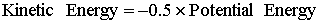40
The potential energy is:41
The kinetic energy is:42
Therefore, from equations 40, 41 and 42 we have:43
This gives: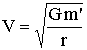44
Let us consider that we displace the orbiting mass by a small radial distance dr. Using the derivative of equation 44, we find that the required corresponding physical change of orbiting velocity dV with respect to the change of radius dr is:45
Substituting 44 in 45 gives:or46
From equation 46, we see that when the number of radial units r decreases (due to energy conservation), Newton's laws require that the relative (number of units of) velocity increases by (minus) half the relative change of radius. Using the principle of mass-energy conservation, we have seen above in equation 38 that the number of Mercury-meters [N-mM] is different from the number of Earth-meters [N-rE] (when measuring the same absolute length). When an observer on Mercury measures the length of his orbit around the Sun, he will measure it using his longer Mercury meter.  Therefore he will find a smaller "number" of meters, than if measured by the Earth observer (who uses the Earth meter).  From equation 38, we find that that smaller number of local meters on Mercury is given by the relationship:
 [N-rm] =(1-1.5e)[N-rE] 47
This variation of the number of meters can also be expressed as a fraction representing the relative change of number of meters (between the Mercury measurement and the Earth measurement) with respect to the total number. Using equation 47, we have:48
The Mercury observer also wishes to measure the change of velocity of his planet using his own units, and compare it with a measurement done by a remote Earth observer.   We have seen in equation 46 that the relative change of velocity is minus half the change of radius.  Using that relationship, let us calculate the relative change of velocity.  Putting 48 in 46 gives: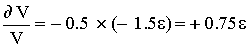49
Let us express that function as the "number" of meters.  The relative change of number of Earth-units of velocity with respect to the number of Mercury-units of velocity, from equation 49 gives:50
Equation 50 can also be written:51
Let us discuss the physical phenomenon implied in equation 51. We know that "locally", Newton's laws of physics are always valid in all frames. For example, if the Earth orbit would drift near the Sun at the Mercury distance, we would still be submitted to exactly the same Newton's equations, using the local units of length and time etc. . ..  existing on Mercury.  Newton equations are always perfectly valid locally inside all frames, on the condition that we use local units.  Therefore the solution presented in this paper will be compatible with that fact.  However, using his local standard units of reference, and newton's laws, his calculation will not be compatible with the fact that there is an advance of the perihelion of the elliptical orbit.  For the Mercury observer, the perihelion of the orbit is not local.  The observer of Mercury must modify the data obtained in his own observation in order to be compatible with the fact that the line passing through the Sun and his own planet is speeping the sky as a function of time.  Therefore the Mercury observer must use the correct (Outer Space) standard units of reference, which are not the standard units existing locally on his frame.
Let us us now consider the length of the corresponding circular orbit.  Since Mercury travels at a higher velocity than previously calculated with Earth units, the time taken by Mercury (period of rotation) around the Sun is reduced. We see in equation 51, that this period of rotation, giving the time taken to complete a rotation of 360 degrees or 2p radians around the Sun is shortened. However, in compatibility with the principle of physical reality, there is a difference between the real length of the trajectory to complete this circumference for an observer using Mercury units and for an observer using Earth units.
We will see here that the advance of the perihelion of Mercury is due to the fact that Mercury actually travels a longer path, (longer than 2pr) before the elliptical orbit is closed (because we have applied Newton's laws combined with Mercury units).  We see below that due to the larger velocity required by Newton's laws, using Mercury units, it takes a shorter time to complete 360 degrees on the circumference of Mercury orbit.  We have shown above in sections 2, 3, 4 and 5, that an ellipse corresponds to an oscillation on each side of the curved axis of a circle.  Using Earth units in both frames, (which is wrong) when calculating the period of oscillation on each sides of the ellipse in equation 31, we found in equation 33, that it takes the same time to complete the oscillation on either sides of the circle than the time to complete the rotation of 360 around the Sun.  However, using the number of Mercury units, corresponding to the same physical length, we will see below that it takes a different time to complete the oscillation on either sides of the circle (equation 31) than the time to complete the rotation of 360 around the Sun.

9 - Taking into Account Mass-Energy Conservation.
Let us apply equation 28 to the classical units existing on Earth. We have seen that the period of oscillation of Mercury on both sides of the average radius ro calculated by an observer on Earth would be calculated as:52
In equation 52, the physical quantities have been substitued by the corresponding "number" of units.  However, this equation cannot be applied without corrections, because this equation uses masses (and all other units) as they exist on Earth, while the interacting mass with the solar gravitational field is different at Mercury orbit (as mentioned above). We recall that the sub index E (as in PE) means that the units used are the ones existing far from the Sun where the principle of mass-energy conservation does not require correction.  From equation 37, we can see that on Earth, the standard meter is shorter than on Mercury. Therefore, the number of Earth-meters [N-rE] is larger than the number of Mercury-meters [N-rM], when measuring the same physical length.  We have: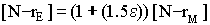53
In equation 39,  we have: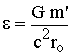54
We know that G is an absolute physical constant. However, since the standard units existing on Mercury are different from the standard units on Earth, different numbers will then express the same physical gravitational constant G.  The gravitational force at one point is physically the same, independently of the units used by the observer.  However, the number of units to describe it will be different, since the reference units are different, but this will represent the same field and the same physical force.  Therefore we have to calculate the relationship giving the change of the number of local units of the gravitational constant G due to the change of standard units on Mercury in compatibility with the principle of mass-energy conservation. The change of the number of units [N-G] of G has been calculated previously ( Chapter 4 Equation 4.65) in the case of zero velocity.  We must then compare the numerical value of G when two conditions are changed simultaneously. One is due to the physical change of velocity as given in equation 51. The other is a mathematical transformation, because we have to express the very same physical quantity using different standard units (from Earth units to Mercury units).
Let us calculate the relationship between the number of gravitational units [N-GM], and [N-GE], resulting from those two changes. This can be done using the relationship giving an equal force between the gravitational force and the centrifugal force. Since the centrifugal force must always be equal to the gravitational force using proper values, we have in all frames, (when using proper units):55
This is mathematically equivalent to:56
Let us use the correct notation showing the number of units. To get the correct physical answer, one must use the number of Mercury-units, since it is the relevant number of units existing at the location where the phenomenon takes place.  The change of the number of units of G can be calculated using the relationship 56 equals to G.  Using the notation, and taking into account the number of units leading to the same quantity, equation 56 should be written:57
Let us compare this number [N-GM] in Mercury units, with the number [N-GE] of units of G on Earth. On Earth, the relevant number of units is:58
Due to the physical change of velocity given by equation 51, we have seen that: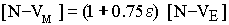59
From equation 37 when we measure the same physical length, we find that the change from the number of Earth-meters [N-rE] to the number of Mercury-meters due only to mass-energy conservation gives: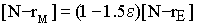60
Since we are using Mercury units, let us now calculate the solar mass. We want to determine the number of Mercury kilograms required to be equal to the mass of the Sun. However, the Mercury kilogram has to be given as a function of the Earth kilogram, which is assumed at rest and sufficiently far from the gravitational potential of the Sun. Therefore, the number of units of Mercury-kilograms will be different from the standard Earth kilogram, because the Mercury kilogram is located deeper in the gravitational potential of the Sun and furthermore because of its velocity.
The change of the number of Mercury kilogram [N-mM] , to measure the solar mass, instead of the Earth kilogram, is calculated in the following way. Due to the gravitational energy, the Mercury kilogram (at rest) is smaller than the Earth kilogram (at rest). Due only to gravity, equation: (see  chapter 4 equation 4.42)gives:(Due only to gravity) 61
Where the indexes (E) and (M) give respectively the location of the mass on Earth or on Mercury. Here those masses are expressed in Mercury units (using the sub-index "M"). In addition, we know from Newton's laws, that the kinetic energy of an orbiting mass around the Sun is half the (negative) gravitational potential energy. Consequently, the kinetic energy of Mercury gives a larger mass on Mercury (expressed in Mercury units) than at rest (always on Mercury):62
Combining equations 61 and 62, we find that the relative mass of a Mercury-kilogram in motion [mM(m)(moving)] with respect to an Earth-kilogram at rest on Earth [mE(E)(rest)] is (expressed in Earth units):63
Therefore, the moving standard Mercury kilogram is slightly less massive than the standard Earth kilogram at rest when common units are used.  However, the quantity required is different. It is the number of Mercury kilograms to equal the mass of the Sun. Of course, the absolute mass of the Sun does not change because it is measured with respect to the Mercury moving kilogram. However, the number of Mercury kilograms that represents the Sun will be different. From equation 63, we can deduce that the number of Mercury-kilograms in the Sun is larger than the number using Earth-kilograms according to:64
We can now calculate the number of gravitational units [N-GM]. Putting 59, 60 and 64 in 57 gives:65
Always neglecting e2 and higher powers of e with respect to e , equation 65 is equal to:66
Using equations 66 and 58, we get:67
We have taken into account that the absolute physical value of the universal gravitational constant G never changes. However, since it is now measured using Mercury units, its numerical value has changed. Furthermore, we have seen that the velocity (equations 51 and 59) of Mercury has changed. Equation 67 has taken these two phenomena into account.
Let us now calculate the period of oscillation on each sides of the radius ro. Substituting 60, 64, and 67 in 52, we get:6869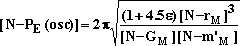70
The period of oscillation of Mercury calculated with Mercury units is then:71
Similarly to equation 52, we can easily formulate the period for the Mercury observer. Combining equation 51 with 71 gives: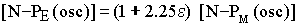72
Equation 72 gives the period of oscillation [N-PM(osc)] of Mercury on either side of the average radius with respect to the initial period of oscillation [N-PE(osc)] using Earth parameters (from infinite distance). We have seen that [N-PE(osc)] is the period when mass-energy conservation is not considered (or when we are at a near infinite distance from the Sun and with near zero velocity). However, since the period of oscillation [N-PE(osc)] without mass-energy corrections is identical to the (initial value of) period of rotation (see equation 33), then equation 72 shows the increase of period with respect to a fixed direction in space.

10 - Relative Distance Reached after One Rotation and One Oscillation.
We have seen in equation 51 that, in order to satisfy Newton's physics with the local parameters, Mercury must travel more rapidly on its orbit according to the relationship: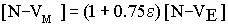73
Even if Mercury travels more rapidly on its orbit, the length of the perimeter of the orbit to complete 360 degrees or 2p radians does not change. Consequently, the length traveled by Mercury to turn 360 degrees around the Sun does not change even if the velocity is increased.
However, we have seen in equation 72 that due to the change from standard Earth units to standard Mercury units, Newton's equations give a longer period of oscillation of Mercury (longer time) on either side of the average radius ro. Due to that longer period of oscillation, Mercury will travel a longer distance before closing the ellipse. Furthermore, since the physical velocity V of Mercury is larger (due to mass-energy conservation) as seen in equation 51, the distance traveled on the orbit becomes longer due to the increase of period, but also due to the increase of velocity. Let us compare the distance traveled by Mercury to complete one full rotation by 360 degrees with the distance traveled to complete an oscillation, which closes an elliptical orbit. The distance traveled when completing one oscillation is:74
We have seen that due to the use of Mercury units and the local agreement with Newton's laws (using proper values), the real physical velocity of Mercury is larger according to equations 51 (and 59). The corrected velocity [N-V(corrected)] with respect to the non-corrected velocity [N-V(non-corrected)] is:75
Similarly, as demonstrated in equation 72, due to the change of standard units on Mercury, the same physical forces measured with the Mercury units, lead to a slower period of oscillation across the average radius ro. This gives: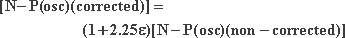76
The correction of length needed to close the elliptical orbit is given by the parameters corrected for mass-energy conservation. Equation 76, written correctly becomes:77
Substituting equations 75 and 76 in 77, we get:78
Taking into account that e is extremely small, we neglect the second order term (e2). Equation 78 becomes:79
This is also equal to:80
Let us note that the relative change of distance of equation 80 (or relative change of angle) is the same whether we use Mercury meters or Earth meters (we neglect higher orders in e2).   The indexes E or m are useless since the increase of angle a is the same in both frames, as expected logically. Equation 80 is illustrated on figure 4.Figure 4

On figure 4, the circumference of the orbit is unrolled at the average radius ro (circle) and forms the "Distance" axis. We have seen that the circumference of the orbit is not changed since Mercury orbits around the Sun is calculated at the same absolute radius ro. However, the real distance traveled by Mercury during one full transverse oscillation is longer than the translation of 360 degrees, due to the slower oscillation on each sides of the radius ro and the increase of velocity resulting from the use of Mercury parameters. This explains the advance of the perihelion of Mercury.
Let us compare equation 76 with Einstein's predictions and astronomical observations. From equations 38 and 80 we get:81
Equation 81 shows that the advance of the perihelion of Mercury, calculated above with a small eccentricity is mathematically identical to equation 5.45 in section 5.10 of .  Without the second order e2 for the eccentricity, in the case of Mercury, this leads to an accuracy of 96%.  Taking into account the second order e2 for the eccentricity, the non-linear change of gravitational energy adds a small correction, which is now taken into account. It has been previously demonstrated  (section 5.10) that the expression (1-e2) must then multiply the denominator of equation 81, because the gravitational potential is not linear between a small and a larger elliptical orbit (taking into account the second and higher order terms). This previously demonstrated phenomenon  (section 5.10) is not repeated here, but it is easily applied to equation 81.
The final equation then gives the change of distance to close an elliptical orbit, with respect to a rotation of 2p radians. Using equation 81, expressed as a function of the angle of precession Df per century, and a larger eccentricity e explained in section ( (section 5.10).  We find that this gives  (equation 5.45):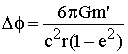82
Equation 82 is mathematically identical to Einstein's equation. Therefore, this shows that the advance of the perihelion of Mercury can be fully predicted using only classical mechanics, without any of Einstein's hypothesis and without space-time distortion. Neither new physics nor any mathematical hypotheses have been used in the above demonstration. Everything is now logical, realistic, and based on mass-energy conservation.

11 -Illustration of the Advance of the Perihelion of Mercury.

Figure 5 illustrates "in perspective" the periodic rotation of Mercury around the Sun. It shows the precessing elliptical orbit of Mercury (dotted curve) oscillating on both sides of the circular orbit at ro (solid line), while the Sun moves uniformly to the right hand side of the figure. The use of the standard Mercury units reduces the number of units representing the force (spring constant) which always pulls Mercury back toward the average radius ro. Therefore, the period of oscillation is longer. Then, the oscillation is not completed (ellipse not closed) when Mercury has already completed a full rotation (360 degrees) around the Sun. We see that the ellipse is crossing the circle upward (at ro) after more than one geometrical rotation of 2p radians. The upward crossing between the elliptical orbit (dotted line) and the circular (solid line) line is repeated only after a supplementary rotation angle a (after each 2p radians).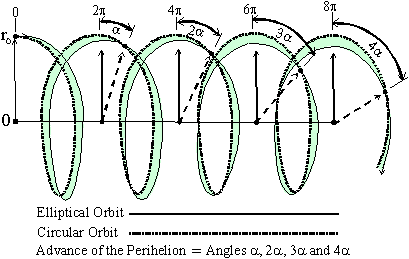Figure 5

Figure 5 shows this cumulative advance a after several rotations. The cause of the advance of the perihelion of Mercury is now perfectly explained. We see that this demonstration has been done using Newton's physical laws instead of non-realistic mathematical models. This paper shows the beauty and the universality of Newton's laws in Galilean coordinates. Those laws are valid internally, everywhere inside all frames when we use proper values. We msut recall that the basic principle of mass-energy conservation has also been foreseen by Newton .
It has been reported that Einstein said: Since the mathematicians have invaded the theory of relativity, I do not understand it anymore . It is the author's wish that the realistic physical explanations presented in this paper will never be drowned into the mathematics.

12 - Acknowledgments.

The author wishes to acknowledge the helpful encouragement of Mr. Bruce Richardson. Stimulating questions were also appreciated from C. Couture, S. Talbert, I. McCausland, T. Phipps and T. Durt.

<><><><><><><><><><><><>

Appendix I
Description of an Ellipse with a Small Eccentricity.

On order to get a physical description of the transformations of a circle into an ellipse with a small eccentricity, we must examine the fundamental properties of ellipses. There is a general belief that a circle becomes an ellipse simply by flattening the circle. It is not realized that for a small eccentricity, an ellipse is much better described as a circle in which the center of forces is shifted away from the center. The flattening of the circle appears only as a second order in a series expansion as demonstrated here. Let us start with a fundamental property of ellipses. One of the definitions of an ellipse  given by Brink is:
An ellipse is defined as the locus of a point the sum of whose distances from two fixed points (the foci) is a constant.
From this property of an ellipse, gardeners and others often construct ellipses by driving pins at the foci and running a loop of string around both pins, leaving a certain amount of slack. A marker P is then placed inside the loop and moved around in such a way as to keep the string taut. Since the amount of slack is constant, the sum of the distances of the marker from the foci is constant, and the marker traces out an ellipse. Such a construction is compatible with the exact definition of an ellipse given by Brink .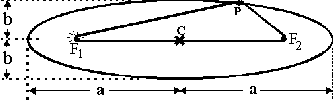Figure 6

Figure 6 shows an ellipse with a large eccentricity. The distance "a" is the semi-major axis and b is the semi-minor axis. The eccentricity e is given by the relationship: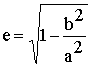A-1
It has also been demonstrated that an elliptical orbit illustrated on figure 6, corresponds exactly to the orbit of a body for which the center of forces (here the Sun at F1) occupies one of the foci. This elliptical shape is the one followed by most comets. Let us examine the way an orbit is gradually transformed from a circle to an ellipse. First, when the eccentricity increases, and before the circular shape of theorbit gets noticeably flatter, the center of forces (focus F1 where the Sun is located) drifts from the center of the original circle C to the left focus at F1. For a large eccentricity, the minor axis 2b then starts to decrease with respect to the major axis 2a, as the square of the displacement of F1.  Figure 6 above illustrates a large eccentricity. In the case of a small eccentricity, this is studied using figure 7.
Figure 7 also illustrates the locus of a moving point P for which the sum of the distances from the two foci (F1 and F2) is constant. When the distance between the two foci is zero, we have a circle and the two foci are superimposed at the same central location. The radius of that circle is R.  Let us move foci F1 to the left-hand side by the amount Dx (from C to F1). We see on figure 7, that when the length of the string is kept constant, (equal to two R) the sum of the distances F1-S1-F2 does not change when Dx increases. The focus F2 moves always in the opposite direction and by the same amount as F1.
We also find the same constant total distance F1-S3-F2 on the left-hand side when the point moving on the ellipse is at S3. In that case, the major axis (in the horizontal direction) of the ellipse is constant (equals 2a) for any eccentricity, when the foci are shifted more apart.Figure 7

Let us now calculate the length of the (vertical) minor axis (2b) as a function of the shift (Dx) of the focus F1. The length of the string passing through F1-S2-F2 is equal to the length F1-S1-F2 (which is equal to the major axis). Consequently, using the triangle F1-S2-F2, formed by the string, the semi major-axis "a" is constant while the semi-minor axis "b", (the distance S2-C), is equal to:A-2
Using a series expansion for ArcSin and Cos, equation A-2 gives: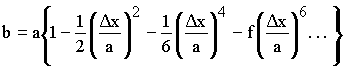A-3
In that series expansion, there exists no term with power one of Dx/a. Since Dx/a is infinitesimal in the problem studied in this paper, the correction for the change of "b" as a function of Dx (which is a quadratic function (second order)) is insignificant. The flattening of the trajectory is observable only for a large value of Dx/a. This shows that for a small displacement Dx of the focus F1 with respect to the center of the orbit, the minor axis does not change appreciably with respect to the major axis and the original orbit remains practically circular, even when the center of forces has moved considerably apart (by Dx), as seen on figure 7.
Let us consider a numerical example. An elliptical orbit, at an average distance of about 50 million of kilometers from the Sun, and having an amplitude of oscillation of one kilometer with respect to the exact circular orbit, implies that the Sun is one kilometer away (value of Dx) from the central circle. From equation A-3, (and other calculations) we can also show that in that case, the major axis has not changed at all and that the change of perimeter of this elliptical orbit, with respect to the circular orbit is surprisingly small. For such an astronomical orbit, for Dx equals one kilometer, the change length of the perimeter is only about 0.01 millimeter. This second order correction is clearly negligible. Of course, for a large eccentricity, the corrections imply other corrections due to the principle of mass-energy conservation. However, they are irrelevant here but they have been taken into account later in this paper in equation 78 and in the original paper on the subject .
This conclusion is also very important because in section 10 we had to calculate the perimeter of an ellipse having a small eccentricity (a first order series expansion). Consequently, we see now that the first order series expansion contains no first power term. Therefore in that case, the perimeter of the ellipse with a small eccentricity is equal to the circumference of the circle.

<><><><><><><><><><><><>

13- References.

[1A] Die Räumliche und zeitliche Ausbreitung der Gravitation.  Von Paul Gerber.  Zeitschrift für Mathematik und Physik Vol.
43,  Pages 93-104 (1898).
Also: Paul Gerber, Die Fortplfanzungsgeschwindigkeit der Gravitation, Annalem der Physik, Vol 52, p. 415-444, 1917 (*)
 P. Marmet, "Einstein's Theory of Relativity versus Classical Mechanics", Ed. Newton Physics Books, 200 pages (1997), Ogilvie Rd. Gloucester, On. Canada, K1J 7N4, also on the internet at the address: http://www.newtonphysics.on.ca/einstein/index.html
 R. P. Feynman, The Feynman Lectures on Physics, Vol. 1, chapter 21, Addison-Wesley Pub, Co. 1963.
 P. Rowland, "Newton and the Concept of Mass-Energy" Department of History, University of Liverpool, Liverpool University Press, P.O. Box 147, Liverpool, L69 3BX (1990).
  Meta Research Bulletin, Editor's note, 3, 1 (11) 1994, P.O. Box 15186, Chevy Chase, MD, 20825-5186, USA.
 R. Brink, Analytical Geometry, Ed. D. Appleton-Century Company Inc. New York, P. 217 (1935).
 P. Marmet, "Absurdities in Modern Physics: A Solution" (1993), Ed. Les Editions du Nordir, c/o R. Yergeau, 165 Waller St. Simard Hall, Ottawa, On. Canada K1N 6N5.
 Sagnac, M. G. J. de Phys. 1914, 4, 177-195.
 A. G. Kelly, "The Sagnac Effect and GPS Synchronisation of Clock-Stations" International Meeting: Galileo Back in Italy, Bologna, Italy, May 26-29 1999.
<><><><><><><><><><><><>

If you read this paper after chapter six of the Book: Einstein's Theory of Relativity versus Classical Mechanics

<><><><><><><><><><><><>
This paper on the Web is a revised and corrected version of the original paper.
---
This paper has been published under the title: "Classical Description of the Advance of the Perihelion of Mercury" in Physics Essays Volume 12, No: 3, 1999,  P. 468-487.
---
This paper has been presented at the International Meeting: "Galileo Back in Italy II" Bologna Italy, 26-30 May 1999,
Title: "Einstein's Mercury Problem Solved in Galileo's Coordinates" .. This paper is printed in the proceedings: "Galileo Back in Italy" Istituto di Chimica, "G. Ciamician",  Via Selmi 2 - Bologna, Italy. P. 352 to 359.
---
This paper has also been presented as an invited speaker at the meeting of the Society for Scientific Exploration  at Albuquerque, June 3-5, 1999.  The title is: "A Logical and Understandable Explanation to the Advance of the Perihelion of Mercury"

<><><><><><><><><><><><>